# Закажи себе WolframAlpha|Pro! Получи пошаговые решения твоих задач!

## ЕГЭ с Wolfram|Alpha :: 28.04.14 :: Вариант 3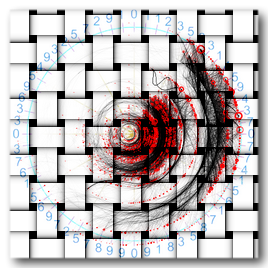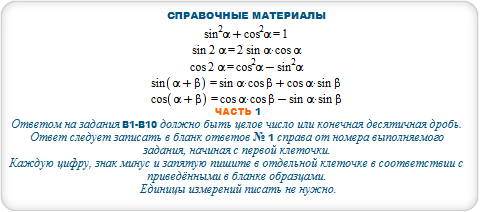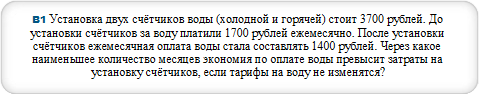#### n>a/(b-c) where a = 3700, b = 1700, c=1400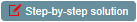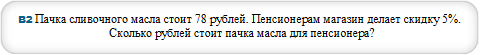#### b = N[a*(1-p/100)] where a=78,p=5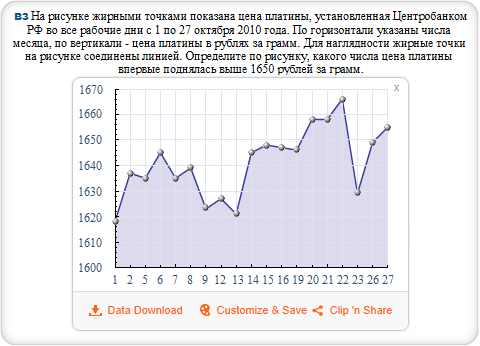#### {18,37,35,45,35,39,23,27,21,45,48,47,46,58,58,66,29,49,55}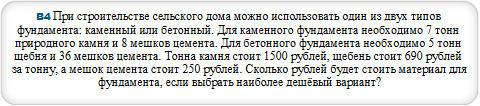#### B=m*v+n*w where m=5,n=36,v=690,w=250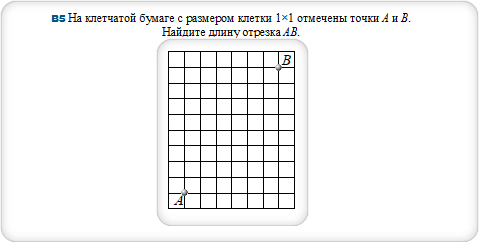#### triangle (0,0),(6,0),(6,8)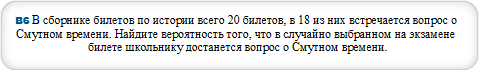#### p=N[m/n] where m = 18, n = 20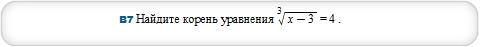#### solve (x-3)^(1/3)=4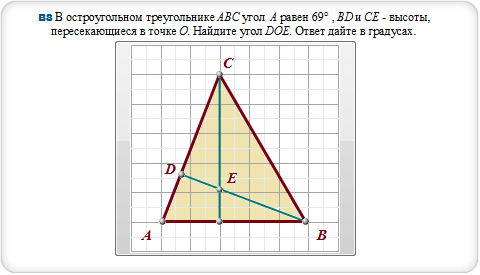#### polygon [0,0],[-3.8,0],[0,10],[5.8,0],[0,0],[0,10],triangle [-3.8,0],[-2.6,3.2],[5.8,0]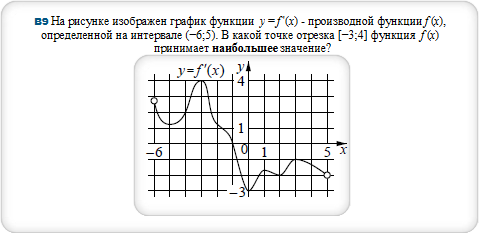#### fit polynomial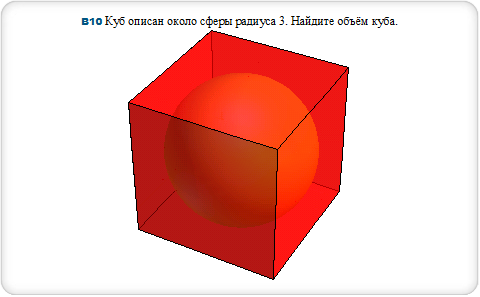#### sphere radius 3, red cube edge length 6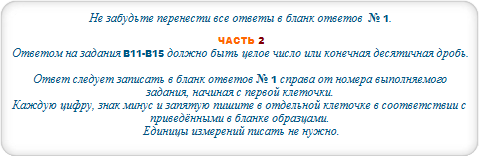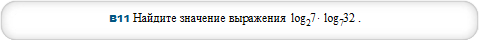#### log(2,7)*log(7,32)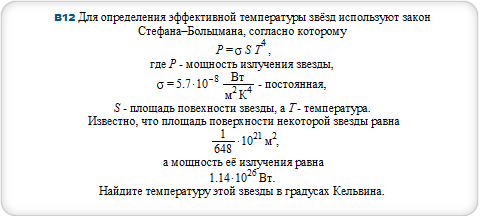#### T = (P/(sigma*S))^(1/4) where P = (114/100)*10^26, sigma = (57/10)*10^(-8), S = (1/648)*10^21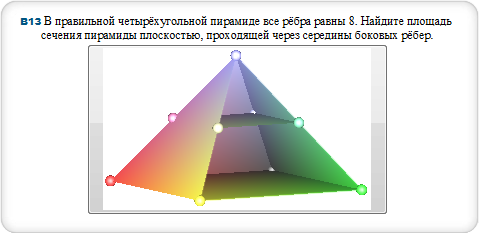#### square pyramid base 8,height 4sqrt2 and red square pyramid base 4,height 2sqrt2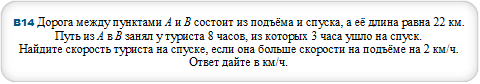#### v = (a*(T-t)+s)/T where s=22,T=8,t=3,a=2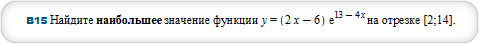#### maximize (2*x-6)*E^(13-4*x) over [2,14]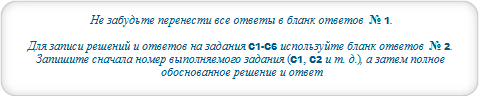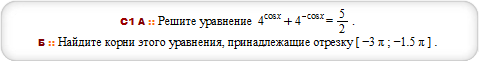#### 4^cos(x)+4^(-cos(x)) = 5/2, x from -3*Pi to -3*Pi/2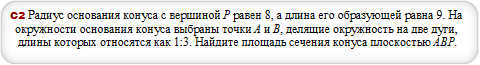#### cone with radius 8, height sqrt17 and triangle (0,-8,0),(-8,0,0),(0,0,sqrt17)#### solve {7^x+98/7^x >= 51,log(x+3,(x+1)/3) <= 0}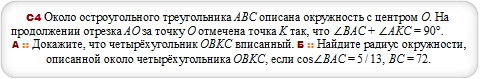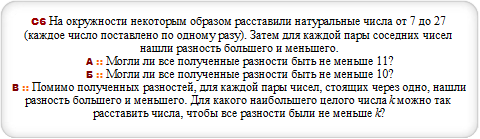#### 7,14,22,8,15,21,9,16,23,10,17,24,11,18,25,12,19,26,13,20,27